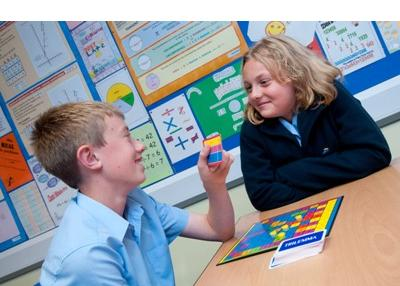# Mathematics

### What will you be learning?

The aims of the course are for students to develop their mathematical skills in a way which encourages confidence as well as providing satisfaction and enjoyment.

Maths is for everyone. It is diverse, engaging and essential in equipping students with the right skills to reach their future destination, whatever that may be. We support students to engage with, explore, enjoy and succeed in maths.

All students have the opportunity to follow a course that enables them to be numerate and builds on what they already know.  This course encourages students to:

• Consolidate their understanding of mathematics
• Be confident in their use of mathematics
• Extend their use of mathematical vocabulary, definitions and formal reasoning
• Develop the confidence to use mathematics to tackle problems in the workplace and everyday life
• Take increasing responsibility for the planning and execution of their work
• Develop an ability to think and reason mathematically
• Learn the importance of precision and rigour in mathematics
• Use a calculator effectively and efficiently
• Make connections between different areas of mathematics
• Realise the application of mathematics in the world around them
• Use ICT appropriately
• Develop a firm foundation for appropriate further study.
• Develop fluent knowledge, skills and understanding of mathematical methods and concepts
• Acquire, select and apply mathematical techniques to solve problems
• Reason mathematically, make deductions and inferences and draw conclusions
• Comprehend, interpret and communicate mathematical information in a variety of forms appropriate to the information and context.

Content

Students will have three or four timetabled lessons each week, each of one hour duration and should expect to spend time on additional study (including homework and reading through notes).  Homework will regularly be set each week and can be up to a total of two hours per week.

The course covers six areas of mathematics:

1. Number
2. Algebra
3. Ratio, proportion and rates of change
4. Geometry and measures
5. Probability
6. Statistics

### What is the assessment?

The scheme of assessment is linear with three question papers at each tier to be taken in the same examination series. The following information is the same for both Foundation and Higher tiers.

• Paper 1: Written paper (non-calculator) – 33⅓% of the GCSE Mathematics assessment
1 hour 30 minutes
80 marks
• Paper 2: Written paper (calculator) – 33⅓% of the GCSE Mathematics assessment
1 hour 30 minutes
80 marks
• Paper 3: Written paper (calculator) – 33⅓% of the GCSE Mathematics assessment

1 hour 30 minutes
80 marks

All papers contain a mix of a mix of question styles, from short, single-mark questions to multi-step problems. The mathematical demand increases as a student progresses through the paper.

### What will you be working towards?

Students will be working towards a GCSE in Mathematics following the GCSE Mathematics curriculum.  Students in Sets 1 and 2 normally following the Higher Tier scheme of work and students in Sets 3, 4 and 5 following the scheme at Foundation Tier.  Students following the Higher Tier course will have access to grades 9 - 4.  Students following the Foundation Tier have access to grades 5 – 1.

Method of Study

It is hoped that you will experience a variety of approaches to study, including:

• Formal class work
• Use of investigations
• Use of group work
• Use of calculators and computers
• Regular internal assessment and feedback to help with your studies.

You will find some topics more difficult than others.  Your teachers will be more than happy to assist you with these difficulties, both in class and outside lesson time, if you ask.

### What happens when you have finished?

The course can lead to further study e.g. A-Levels, National Diplomas, access courses for mature students, training and employment.  A grade 5 at GCSE is required for many numerical related courses and jobs and is particularly useful for running a business, computer courses, handling household accounts etc.